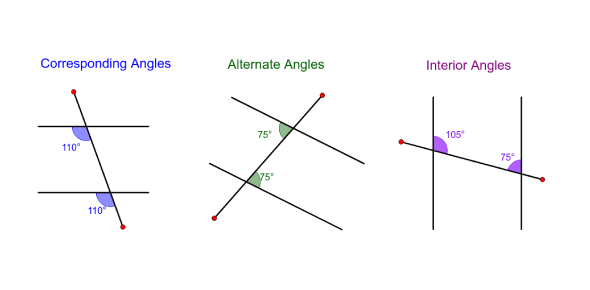# Angle Properties - Parallel Lines

16 Questions | Total Attempts: 10627SettingsPractice skills and knowledge of the relationships of angles on parallel lines and transverses.

• 1.
Angles a and b are:
• A.

Alternate

• B.

Co-interior

• C.

Vertically-opposite

• D.

Corresponding

• E.

Exterior

• 2.
Angles a and c are:
• A.

Alternate

• B.

Co-interior

• C.

Vertically-opposite

• D.

Corresponding

• E.

Exterior

• 3.
Angles b and c are:
• A.

Alternate

• B.

Co-interior

• C.

Vertically-opposite

• D.

Corresponding

• E.

Exterior

• 4.
Angles b and g are:
• A.

Alternate

• B.

Co-interior

• C.

Vertically-opposite

• D.

Corresponding

• E.

Exterior

• 5.
How many degrees is angle a?
• 6.
How many degrees is angle a?
• 7.
How many degrees is angle c?
• 8.
What is the size of angle g?
• A.

70°

• B.

110°

• 9.
How many degrees is angle g?
• A.

125

• B.

180

• C.

135

• D.

90

• E.

55

• 10.
What is the size of angle d?
• A.

55°

• B.

90°

• C.

125°

• D.

180°

• E.

25°

• 11.
How many degrees is angle f?
• 12.
How many degrees is angle b?
• 13.
How many degrees is angle a?
• A.

180°

• B.

40°

• C.

200°

• D.

140°

• E.

50°

• 14.
How many degrees is angle a?
• A.

60°

• B.

120°

• C.

180°

• D.

160°

• E.

80°

• 15.
How many degrees is angle c?
• 16.
How many degrees is angle d?
Related TopicsBack to top